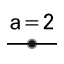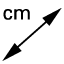# Construction 09: Triangle Inequalities

## Objective:

Explore the Triangle Sides Inequality. Demonstrate the Inequality in one triangles Explore the Hinge Theorem: inequalities in two triangles.

## 9.1 Triangle Inequality: Possible Triangles:

Which of the following could be the lengths of the sides of a triangle?

Select all that apply
• A
• B
• C
• D
• E

## 9.2 Inequalities in One Triangle

Construct a Triangle using a slider for at least one side. 1. Useto create a slider. 2. Create the base of the triangle usinga segment with that given length. (Hint: Use the variable.) 3. Create a triangle 4. Measure all sides and angles.

## Inequalities in One Triangles

As you increase the base, using the slider, how does the angle opposite increase or decrease? Write a general rule for the relationship relative size between opposite angles and sides.

## 9.3 The Hinge Theorem

Explain why this construction guarantees that segments and are congruent and that and are congruent.

## 9.3 The Hinge Theorem

1.Draw two concentric circles. Concentric circles have the same center and difference radii. 2.Draw two segments from center to the circumference of each circle. 3.Measure each side and angle.